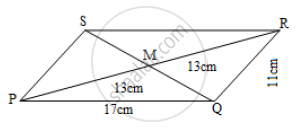# Adjacent sides of a parallelogram are 11 cm and 17 cm. If the length of one of its diagonal is 26 cm, find the length of the other - Geometry

Adjacent sides of a parallelogram are 11 cm and 17 cm. If the length of one of its diagonal is 26 cm, find the length of the other.

#### Solution

Let □PQRS be a parallelogram.Then, PQ = 17 cm, QR = 11 cm and diagonal PR = 26 cm The diagonals of a parallelogram bisect each other. Point M is the point of intersection of diagonals PR and QS.

therefore PM=MR=1/2PR=1/2xx26

therefore PM=MR=13 cm ......(1)

QM=1/2QS

thereforeQS=2QM......(2)

In ΔPQR, QM is the median.

PQ^2+QR^2=2PM^2+2QM^2 .......(By Apollonius theorem)

(17)^2+(11)^2=2(13)^2+2QM^2

therefore 289+121=2(169)+2QM^2

therefore 410=2(169)+2QM^2

Diving by 2, we get

205 =169 + QM^2

therefore QM^2= 205 -169=36

therefore QM=6

therefore QS=2QM=2xx6=12cm

Thus, the length of the other diagonal is 12 cm.

Concept: Appolonius Theorem
Is there an error in this question or solution?